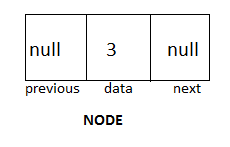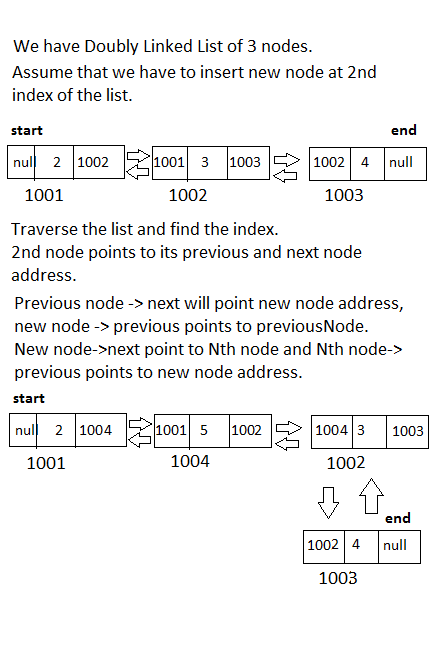As we have already discussed about Linked-List concepts and one of its type "Singly Linked List" in detail in previous topic.

Today I am going to discuss other type of Linked List - Doubly Linked-List and its operations with example.

In Doubly Linked-List each node contains data value two address blocks "previous and next".

Previous points address of previous node and next points address of next node.

It gives tight coupling between nodes.

While traversing list we can traverse it in both directions.

Insertion and Deletion process becomes much easier then singly-linked list.Doubly Linked List Node Definition. ByTechAchievers.com

Doubly Linked List Operations - Implementation

Insertion :

With any new insertion process, new node will create.

Initially Each node will have data value and null value in previous and next block.

We are naming first node of list is START and last node as END.

Initially start and end both will be null.

Assuming createNode is a method that is creation new node for the list.

If start = null i.e. there is list is empty so that new node would become start

and end of the list.
``````
start = null;

end = null;

node = createNode();

if ( start == null ) {

start = node;

end = node;

}``````
Insertion from start : -

If node is not the first node of the list, as we have to insert node from start so that start of the list will change and end will remain same.

start -> previous will have new node's address and new node -> next will have start address.

New node will become new start of the list.
``````
else {

start -> previous = node;

node -> next = start;

start = node;

} ``````Doubly Linked List - Inserting from start. ByTechAchievers.com

Insertion from end : -

If node is not the first node of the list, as we have to insert node from end so that end of the list will change and start will remain same.

End -> next will have new node's address and new node's previous will have end address.

New node will become new end of the list.
``````
else {

end -> next = node;

node -> previous = end;

end = node;

}``````Doubly Linked List - Inserting from end. ByTechAchievers.com

Insertion at any index : -

When we want to insert node at any index, new node will create its space in between two nodes.

Assume that the index is N.

Traverse the list and reach to N index, as we know in doubly linked list every node points to its previous node and next node of the list.

This is the shuffling of node's address, there are mainly three nodes that will do shuffling, previous node , nth node and new node.

Previous node ->next will point to new node address, New node coming in between so that new node -> previous will point to previous node.

New node ->next will point to Nth node address and Nth node ->previous will point to new node.
``````
else {

search_Index();

previous_node = nth_node ->previous;

previous_node ->next = new_node;

new_node ->previous = previous_node;

new_node ->next = nth_node;

nth_node ->previous = new_node;

}
``````Doubly Linked List - Inserting at any index. ByTechAchievers.com

Deletion :

Deletion is the process where we remove node from list.

Same as insertion we will see all three ways to delete node.

Before deletion first will check either the list is empty or not.

If List is empty then will display message.
``````
if ( start == null ) {

display "List is empty, Can not remove node"

}
``````

Deletion from start : -

As we have to remove node from start, every start next points to next node of the list so that start ->next address would become the new start of the list. New start's previous will be set as null.

``````
else {

start = start -> next;

start ->previous = null;

}
``````

Deletion from end : -

As we have to remove node from end, every end previous points to previous node of the list so that end ->previous address would become the new end of the list.

New end's next will be set as null.

``````
else {

end = end -> previous;

end ->next = null;

}``````

Deletion from any index : -

Deletion from any index means removing specific node.

Assume that we have to remove Nth node from the list.

First traverse the list and search that Nth node, When we remove Nth node then its previous and next node will point each other.

``````
else {

search_nth_node();

previous_node = nth_node ->previous;

next_node = nth_node -> next;

previous_node ->next = nth_node ;

nth_node ->previous = previous_node;

}``````Doubly Linked List - Deleting Process. ByTechAchievers.com

Traverse List : -

Traversing means reading nodes values from list. In doubly linked list we can traverse the list in both directions.

In forward direction every node will point to it next node and in backward direction each node will point to its previous node.

Traversing In Forward Direction : -

While traversing make sure that list is not empty, as we are going to read nodes in forward direction so that start should not be null.

Put start value into a temporary variable called Tempwhile reading nodes temp will represent every node, so that start and end should not be impacted.
``````
if ( start != null ) {

temp = start;

while ( temp !=null ) {

System.out.println(" Data Value : - "+temp.data);

temp = temp ->next;

}

}
``````

Traversing In Backward Direction : -

While traversing make sure that list is not empty, as we are going to read nodes in backward direction so that end should not be null.

Put end value into a temporary variable called Tempwhile reading nodes temp will represent every node, so that start and end should not be impacted.
``````
if ( end != null ) {

temp = end;
while ( temp !=null ) {

System.out.println(" Data Value : - "+temp.data);

temp = temp -> previous ;

}

}
``````

Doubly Linked List Implementation - Java Example
``````
class ````Node ````{

Integer data;

Node next;

Node previous;

private static Node start = null;

private static Node end = null;

private static  Node temp = null;

private static Node previousNode = null;

private static Node nextNode = null;

````Node (Integer aData, Node aNext , Node aPrevious) {````

this.next = aNext;

this.previous = aPrevious;

```` }````

````public static void insertionFromBeginning(Node aNode){````

if ( start == null ){

System.out.println("First Node Creation ...\n");

start = aNode;

end   = aNode;

}

else{

aNode.next = start;

start.previous = aNode;

start = aNode;

}

````}````

```` public static void insertionFromEnd(Node aNode){````

end.next = aNode;

aNode.previous = end;

end = aNode;

````}````

``````public static void insertionAtAnyIndex(Node aNode, int index){
``````

int count = 1;

temp = start;

if (start != null ) {

while (temp!=null){

if ( index == 1 ) {

insertionFromBeginning(aNode);

}

if ( count == index ) {

previousNode = temp.previous;

nextNode = temp.next;

previousNode.next = aNode;

aNode.previous = previousNode;

aNode.next = nextNode;

nextNode.previous = aNode;

}

count ++;

temp = temp.next;

}

}

````}````

````public static void deletionFromBeginning(){````

if(start == null || end == null){

System.out.println(" List is empty");

}

else{

start = start.next;

start.previous = null;

}

``````}

public static void DeletionFromEnd(){``````

if(start == null || end == null){

System.out.println(" List is empty");

}

else{

end = end.previous;

end.next = null;

}

`````` }

public static void deletionAtAnyIndex(int index){``````

int count = 1;

temp = start;

if (start != null ) {

while (temp!=null){

if ( index == 1 ) {

deletionFromBeginning();

}

if ( count == index ) {

previousNode = temp.previous;

nextNode = temp.next;

System.out.println( previousNode);

System.out.println( nextNode);

previousNode.next = nextNode;

nextNode.previous = previousNode;

}

count ++;

temp = temp.next;

}

}

``````}

public static void traverseList(char ch){
``````

if ( start == null ){

System.out.println(" List is empty ...");

}

else{

if ( ch =='F')

temp = start;

if(ch == 'B')

temp = end;

while (temp!=null) {

System.out.println( "Node Value - "+temp.data);

if ( ch =='F')

temp = temp.next;

if ( ch == 'B')

temp = temp.previous;

}

}

```` }````
````}````

public class ````DoublyLinkedListExample````{

````public static void main(String []args){````

System.out.println(" \nInsertion from beginning\n ");

Node.insertionFromBeginning ( new Node (1,null,null));

Node.insertionFromBeginning ( new Node (2,null,null));

Node.insertionFromBeginning ( new Node (3,null,null));

System.out.println(" \nTraverse List - process\n ");

Node.traverseList('F');

System.out.println(" \nInsertion from end\n ");

Node.insertionFromEnd( new Node (4,null,null));

Node.insertionFromEnd( new Node (5,null,null));

Node.insertionFromEnd( new Node (6,null,null));

Node.insertionFromEnd( new Node (7,null,null));

System.out.println(" \nTraverse List - process\n ");

Node.traverseList('F');

System.out.println(" \nInsertion at N index\n ");

Node.insertionAtAnyIndex ( new Node (8,null,null),2);

System.out.println("\nTraverse List - process\n ");

Node.traverseList('F');

System.out.println("\nDeletion - process from beginning\n ");

Node.deletionFromBeginning();

System.out.println("\nTraverse List - process\n ");

Node.traverseList('F');

System.out.println("\n Deletion from N Index\n");

Node.deletionAtAnyIndex(2);

System.out.println("\nTraverse List- process\n ");

Node.traverseList('F');

System.out.println("\n Deletion from end \n");

Node.DeletionFromEnd();

System.out.println("\n Traverse List process in forward\n ");

Node.traverseList('F');

System.out.println(" \nTraverse List - process in backward\n ");

Node.traverseList('B');

````}````

}
``````
``````
OUTPUT  -

``````Insertion from beginning
``````
First Node Creation ...

``````Traverse List - process
``````

Node Value - 3

Node Value - 2

Node Value - 1

````Insertion from end````

````Traverse List - process ````

Node Value - 3

Node Value - 2

Node Value - 1

Node Value - 4

Node Value - 5

Node Value - 6

Node Value - 7

````Insertion at N index````

````Traverse List - process````

Node Value - 3

Node Value - 8

Node Value - 1

Node Value - 4

Node Value - 5

Node Value - 6

Node Value - 7

````Deletion - process from beginning````

````Traverse List - process````

Node Value - 8

Node Value - 1

Node Value - 4

Node Value - 5

Node Value - 6

Node Value - 7

````Deletion from N Index````

````Traverse List- process````

Node Value - 8

Node Value - 4

Node Value - 5

Node Value - 6

Node Value - 7

````Deletion from end````

````Traverse List process````

Node Value - 8

Node Value - 4

Node Value - 5

Node Value - 6

````Traverse List - process in backward````

Node Value - 6

Node Value - 5

Node Value - 4

Node Value - 8
``````